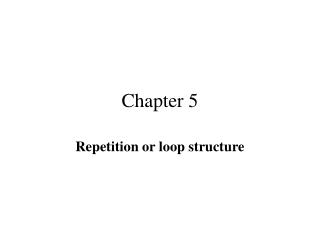Download PresentationChapter 5

# Chapter 5 - PowerPoint PPT PresentationDownload Presentation## Chapter 5

- - - - - - - - - - - - - - - - - - - - - - - - - - - E N D - - - - - - - - - - - - - - - - - - - - - - - - - - -
##### Presentation Transcript

1. Chapter 5 Repetition or loop structure

2. What is repetition or loop? • repeat the execution of one or a group (block; instruction enclosed in a pair of braces) of instructions a certain number of times.

3. Why repetition or loop? • better understood by a simple example: the hourly payroll program introduced earlier computes the pay of only one employee! Yet in general, a company has many employees. • Can you think of other examples?

4. The repetition instructions • There are three different instructions you can choose from: the while instruction, the for instruction, and the do-while instruction. • Which one to choose? (will discuss later.)

5. The while loop • format: while (logical expression) { loop task } • the logical expression is similar to logical expression you saw in if-else structure discussed in chapter 4.

6. Simple hourly payroll program • problem specification: user is prompted to enter # employees. For each employee, the user is asked to enter hours worked and hourly rate. The program then computes and prints out the pay for each employee until. The process repeats until all employees are processed. • Let's work it out together!

7. A program that computes the average of a list of scores • sometime it may not be practical to ask the user to enter how many scores are there (e.g., if the number of scores or students who tool the test are large, it is very time-consuming to count how many scores are there; the manual counting process is error-prone, too.) • A simple solution is to use a sentinel value which is also called a trailer. • Let's work out the program together. How many variables need to be declared? Remember accumulator and counter?

8. The rocket count-down problem • output: 10 9 8 7 6 5 4 3 2 1 Blast off! • Can you work out the program? Let give it a try.

9. Another simple example • Add integers from 1 to 100. • Add integers from n1 to n2. • Add odd integers from 1 to 99. • Add odd integers from n1 to n2. • Add even integers from 1 to 99. • Add even integers from n1 to n2.

10. How about the temperature conversion table Fahrenheit Celsius 32 0.00 31 … … … … … 212 100.00

11. How about tables for math functions • sin(x) • cos(x) • etc.